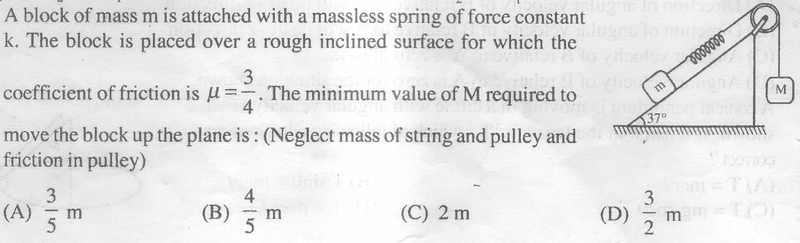# Wedge-Spring-Block ProblemI can't figure out how the answer is (A). The spring is the problem. I thought the spring would just extend and whole thing would act like a string.

Have you learned about gravitational potential energy and spring energy yet?

haruspex
Homework Helper
Gold Member
2020 Award
I thought the spring would just extend and whole thing would act like a string.
I agree, and since k does not appear in any of the answers, the questioner agrees the spring is irrelevant.
My guess is that the coefficient was supposed to be 1/4.

Even when the gravitational force exerted on the block M is equal to the spring force exerted on block M, the block is still moving. Your method presumes that this is not true. If you use conservation of energy to solve for the maximum spring force, answer (A) can be arrived at.

Last edited:
•DSM_
haruspex
Homework Helper
Gold Member
2020 Award
Even when the gravitational force exerted on the block M is equal to the spring force exerted on block M, the block is still moving. Your method presumes that this is not true. If you use conservation of energy to solve for the maximum spring force, answer (A) can be arrived at.
You are assuming that block M is released with the spring just taut, i.e. at its relaxed length. Yes, that gives answer A, so that is probably what is intended, but I see nothing in the problem statement to justify that assumption.

•DSM_
Okay, I have to do it with energy conservation.
If block is released when spring is relaxed, if it moves x downwards,
Work done by grav = Potential energy of Spring.
1/2kx^2 = Mgx
kx = 2Mg
Then tension is kx everywhere in string. Then equating to (friction + mgsin37) gives answer (A).

Is this correct?

haruspex
Homework Helper
Gold Member
2020 Award
Okay, I have to do it with energy conservation.
If block is released when spring is relaxed, if it moves x downwards,
Work done by grav = Potential energy of Spring.
1/2kx^2 = Mgx
kx = 2Mg
Then tension is kx everywhere in string. Then equating to (friction + mgsin37) gives answer (A).

Is this correct?
Seems so. Still a poorly worded question, and congratulations to AlephNumbers for guessing what was meant.

Seems so. Still a poorly worded question, and congratulations to AlephNumbers for guessing what was meant.

I think questioner want us to assume that the spring is relaxed otherwise there wouldn't be any spring at all.

Yup, thanks AlephNumbers :)